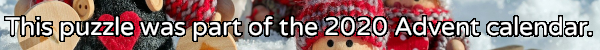mscroggs.co.uk
mscroggs.co.uksubscribe

# Puzzles

## 24 DecemberThere are six ways to put two tokens in a 3 by 3 grid so that the diagonal from the top left to the bottom right is a line of symmetry:
Today's number is the number of ways of placing two tokens in a 29 by 29 grid so that the diagonal from the top left to the bottom right is a line of symmetry.

## 23 December198 is the smallest number that is equal to 11 times the sum of its digits.
Today's number is the smallest number that is equal to 48 times the sum of its digits.

## 22 DecemberPut the digits 1 to 9 (using each digit exactly once) in the boxes so that the sums are correct. The sums should be read left to right and top to bottom ignoring the usual order of operations. For example, 4+3×2 is 14, not 10. Today's number is the largest number you can make with the digits in the red boxes.
 + + = 18 + + + ÷ - = 1/2 + + + + ÷ = 3/2 =24 =8 =13
Tags: numbers, grids

## 21 DecemberThere are 3 ways to order the numbers 1 to 3 so that no number immediately follows the number one less that itself:
• 3, 2, 1
• 1, 3, 2
• 2, 1, 3
Today's number is the number of ways to order the numbers 1 to 6 so that no number immediately follows the number one less that itself.

## 20 December18 can be written as the sum of 3 consecutive (strictly) positive integers: 5 + 6 + 7.
18 can also be written as the sum of 4 consecutive (strictly) positive integers: 3 + 4 + 5 + 6.
18 is in fact the smallest number that can be written as the sum of both 3 and 4 consecutive (strictly) positive integers.
Today's number is the smallest number that can be written as the sum of both 12 and 13 consecutive (strictly) positive integers.
Tags: numbers, sums

## 17 DecemberPut the digits 1 to 9 (using each digit exactly once) in the boxes so that the sums and product are correct. Today's number is the product of the numbers in the red boxes.
 + + = 16 + + + + + = 8 + + + × × = 288 =11 =14 =20
Tags: numbers, grids

## 15 DecemberWhen talking to someone about this Advent calendar, you told them that the combination of XMAS and MATHS is GREAT. They were American, so asked you if the combination of XMAS and MATH is great; you said SURE. You asked them their name; they said SAM.
Each of the letters E, X, M, A, T, H, S, R, U, and G stands for a different digit 0 to 9. The following sums are correct:
Today's number is SAM. To help you get started, the letter T represents 4.

## 14 DecemberThe numbers 33, 404 and 311 contain duplicate digits. The numbers 120, 15 and 312 do not.
How many numbers between 10 and 999 (inclusive) contain no duplicate digits?

## Archive

Show me a random puzzle
▼ show ▼